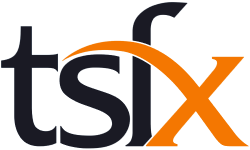# Mathematical Methods Course Catalogue

Mathematics is the study of function and pattern in number, logic, space and structure, and of randomness, chance, variability and uncertainty in data and events. It is both a framework for thinking and a means of symbolic communication that is powerful, logical, concise and precise. Mathematics also provides a means by which people can understand and manage human and natural aspects of the world and inter-relationships between these. Essential mathematical activities include: conjecturing, hypothesising and problem posing; estimating, calculating and computing; abstracting, proving, refuting and inferring; applying, investigating, modelling and problem solving.

Mathematical Methods Units 1 and 2 are completely prescribed and provide an introductory study of simple elementary functions, algebra, calculus, probability and statistics and their applications in a variety of practical and theoretical contexts. They are designed as preparation for Mathematical Methods Units 3 and 4 and cover assumed knowledge and skills for those units.

Mathematical Methods Units 3 and 4 are completely prescribed and extend the study of simple elementary functions to include combinations of these functions, algebra, calculus, probability and statistics, and their applications in a variety of practical and theoretical contexts. They also provide background for further study in, for example, science, humanities, economics and medicine.

## Course List

To filter the course list, enter your search text into the below field

For example, entering Unit 3 into the search field would show all Unit 3 Mathematical Methods Courses.
\Learnworlds\Codeneurons\Pages\ZoneRenderers\CourseCards